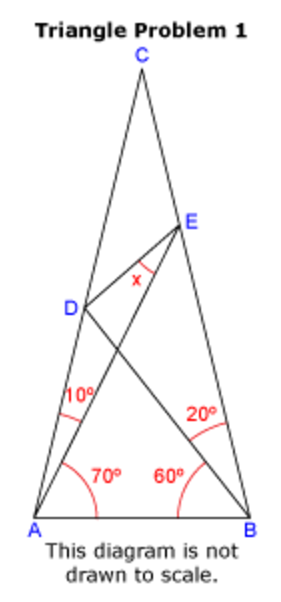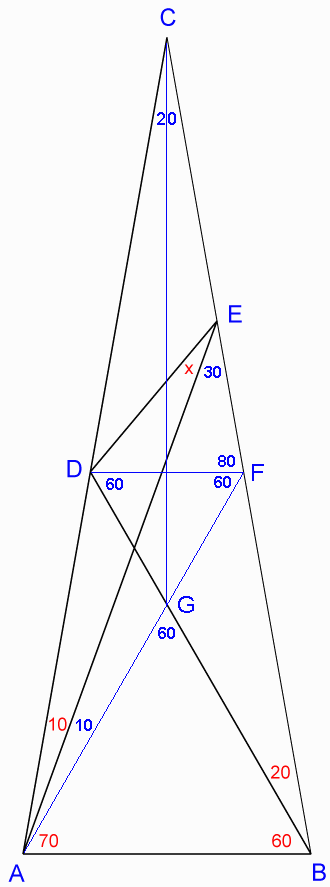D

#### World's hardest easy Geometry problem?

1261 viewed last edited 1 year agoAnthony Schwartz
1I came across this site - http://thinkzone.wlonk.com/MathFun/Triangle.htm . And I have no idea how to solve either of the problems described there. Apparently, this can be done using only elementary geometry. Does anybody have any idea where to start?Sangeetha Pulapaka
11. Calculate some known angles: \angleACB = 180-(10+70)-(60+20) = 20° \angleAEB = 180-70-(60+20) = 30° 2. Draw a line from point D parallel to \overline{AB} labeling the intersection with \overline{BC} as a new point F and conclude: \DeltaDCF\sim\Delta ACB \angleCFD = \angleCBA = 60+20 = 80° \angleDFB = 180-80 = 100° \angle CDF =\angleCAB = 70+10 = 80° \angleADF = 180-80 = 100° \angleBDF = 180-100-20 = 60° 3. Draw a line \overline{FA} labeling the intersection with \overline{ DB} as a new point G and conclude: \DeltaADF\cong\Delta BFD \angleAFD = \angleBDF = 60° \angleDGF = 180-60-60 = 60° =\angle AGB \angleGAB = 180-60-60 = 60° \DeltaDFG (with all angles 60°) is equilateral \DeltaAGB (with all angles 60°) is equilateral 4. \DeltaCFA with two 20° angles is isosceles, so \overline{FC} =\overline{ FA} 5. Draw a line \overline{CG}, which bisects\angle ACB and conclude: \DeltaACG\cong\Delta CAE \overline{FC}-\overline{CE} = \overline{ FA} - \overline{AG}= \overline{FE}= \overline{FG} \overline{FG} =\overline{FD} so \overline{ FE} = \overline{FD} 6. With two equal sides, \DeltaDFE is isosceles and conclude: \angleDEF = 30 ^\circ+x = (180-80)/2 = 50^\circ Answer: x = 20°Sangeetha Pulapaka
0
Click on the figure and open it in a new tab to get the enlarged picture